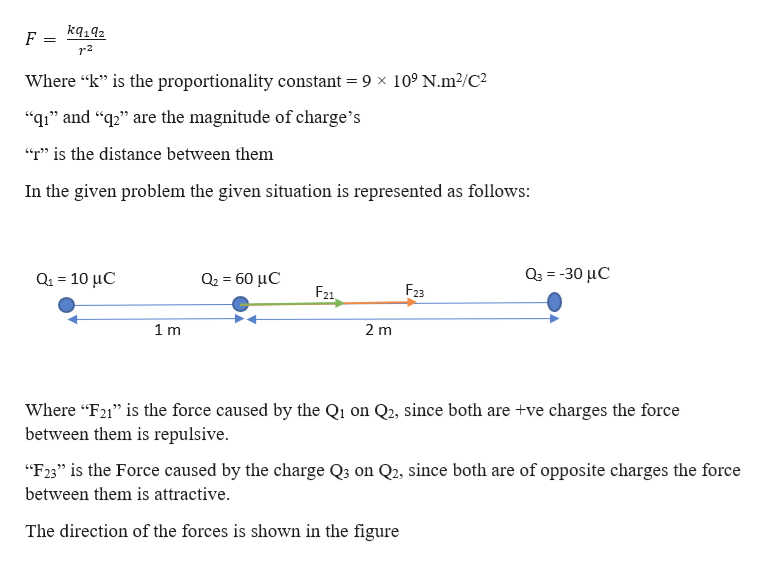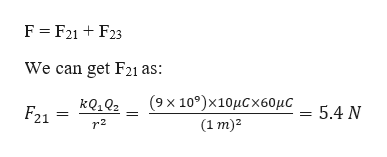# Three-point charges are located on the x-axis. The first charge, q1 = 10.0 uC (micro C), is at x = -1.00 m the second charge, q2 = 60.0 uC (micro C), is at the origin, and the third charge, q3 = -30.0 uC (micro C), is located at x = 2.00 m. What is the force on q2?

Question

Three-point charges are located on the x-axis. The first charge, q1 = 10.0 uC (micro C), is at x = -1.00 m the second charge, q2 = 60.0 uC (micro C), is at the origin, and the third charge, q3 = -30.0 uC (micro C), is located at x = 2.00 m. What is the force on q2

check_circle

Step 1

Given information:

Charge of the first charge (q1) = 10 µC

Location of the charge on x-axis (x1) = -1.00 m

Charge of the 2nd charge (q2) = 60 µC

Location of charge on x-axis (x2) = 0.00

Charge on the 3rd charge (q3) = -30 µC

Location of charge on x-axis (x3) = 2.00 m

Step 2

From coulomb’s law the force acting between two-point charges is given as:help_outlineImage TranscriptioncloseF = r2 Where "k" is the proportionality constant = 9 x 10° N.m2/C2 "q1" and "2" are the magnitude of charge's "r" is the distance between them In the given problem the given situation is represented as follows: ο-30 μC α- 10 μC α-60 μC F23 F21 1 m 2 m Where "F21 is the force caused by the Q1 on Q2, since both are +ve charges the force between them is repulsive. "F23" is the Force caused by the charge Q3 on Q2, since both are of opposite charges the force between them is attractive The direction of the forces is shown in the figure fullscreen
Step 3

From the figure we can write the force act...help_outlineImage TranscriptioncloseF F21F23 We can get F21 as: (9x 10)x10μCx60μC - 5.4 Ν F21 (1 m)2 r2 fullscreen

### Want to see the full answer?

See Solution

#### Want to see this answer and more?

Solutions are written by subject experts who are available 24/7. Questions are typically answered within 1 hour.*

See Solution
*Response times may vary by subject and question.
Tagged in

### Electric Charges and Fields